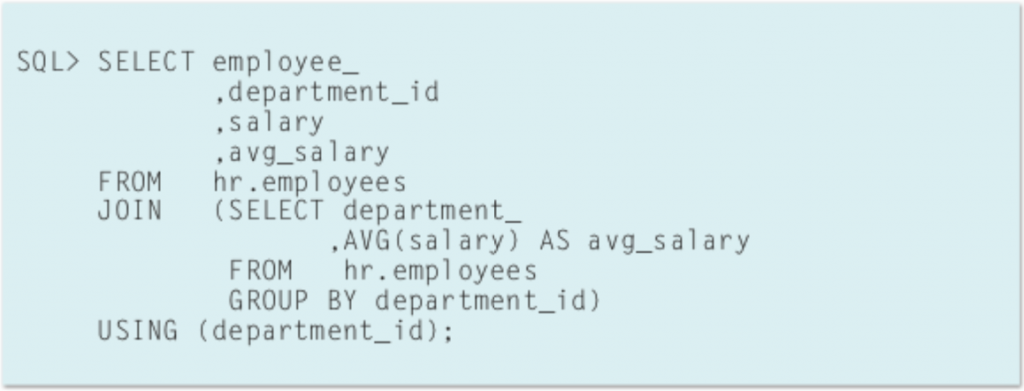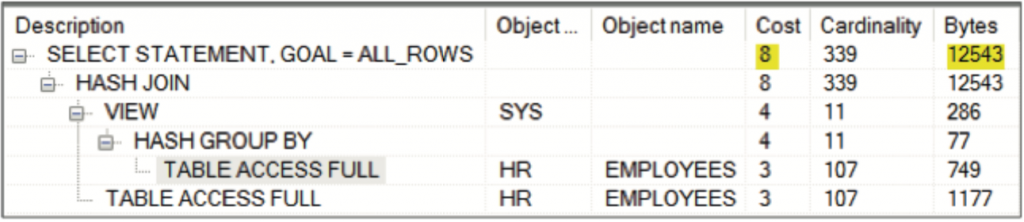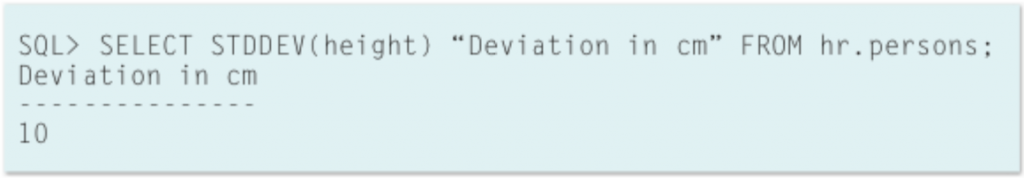• Post category:Database

# Excerpt from Oracle analytic functions: tips and tricks

Infolob’s lead Oracle DBA, Ravi Kumar, recently co-authored an article in OraWorld with Mari Kupatadze of FlashGrid detailing some tips for utilizing analytic functions with Oracle databases in order to cut down on processing times. The more data you can process with the fewest resources, the more efficiently your business can operate, and analytical functions can greatly simplify the process.

I’ve included some excerpts from Ravi and Mari’s article:

The same data can be retrieved in several ways and the best method is chosen based on the following characteristics:

• Optimal usage of resources
• Fast response time
• Code simplicity

Analytical functions give us the opportunity to write shortcode, use resources optimally and get the response quickly.

## Plan Comparison

If we compare execution plans between Analytic and non-Analytic SQL statements, we see its advantage. For example, the following query reports the salary for each employee, along with the average salary of the employees within the department.

##The same SQL statement without analytic function:As you can see, the second code is longer and not easily understandable. Moreover, the plan of the first query shows that retrieving the same set of data requires fewer resources to be used than in the second query.

Mari and Ravi go into quite a bit of detail about analytic and aggregate functions, so if you’d like to take a look at those and read the entire article, it’s available here. I wanted to include their example for the STDDEV function, though, because I found it the most interesting.

## STDDEV:

You may have already heard about the function of STDDEV. The Standard Deviation is a measure of how spread out numbers are. In statistics, it is represented by the Greek letter sigma (?) or the Latin letter s.

Real-World Example:

The Standard Deviation will help you know your height is low, high or medium based on the data that was collected in your country or in the world.

If your height satisfies the following condition you have normal height, otherwise you are tall or small:

v_Mean – v_Standard_Deviation < = You_height < = v_Mean + v_Standard_Deviation

The following picture shows which heights are within one Standard Deviation of the Mean.The formula is easy: it is the square root of the Variance.

## So what is the Variance?

The Variance is an Analytic Function and is the average of
the squared differences from the Mean. It becomes more complicated when you need to calculate STDDEV based on a large amount of data. In the past, when there was no Oracle or other software that has these built-in functions, it would be necessary to manually calculate the Standard Deviation. In that case, first, you need to find a Variance.

To calculate Variance:

• Need to find Mean.
• For each number, subtract the Mean and square the result.
• Work out the average of those squared differences.

When you get the value for Variance, take the square root of it and you get Standard Deviation. Instead of calculating all these values separately, use the Oracle function STDDEV, it is easy.Again, don’t forget to check out the entire article that Ravi and Mari wrote. Both authors are Oracle Certified Masters and their article is full of valuable information about analytical functions.